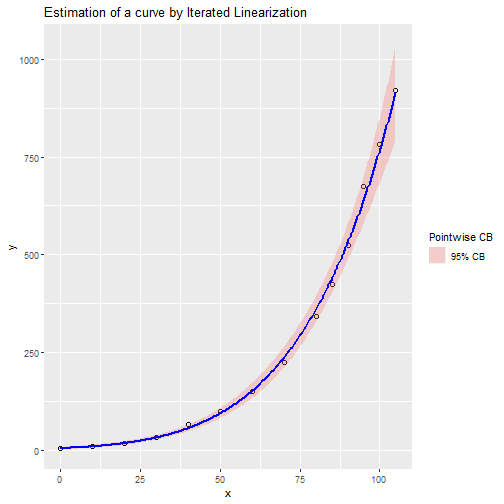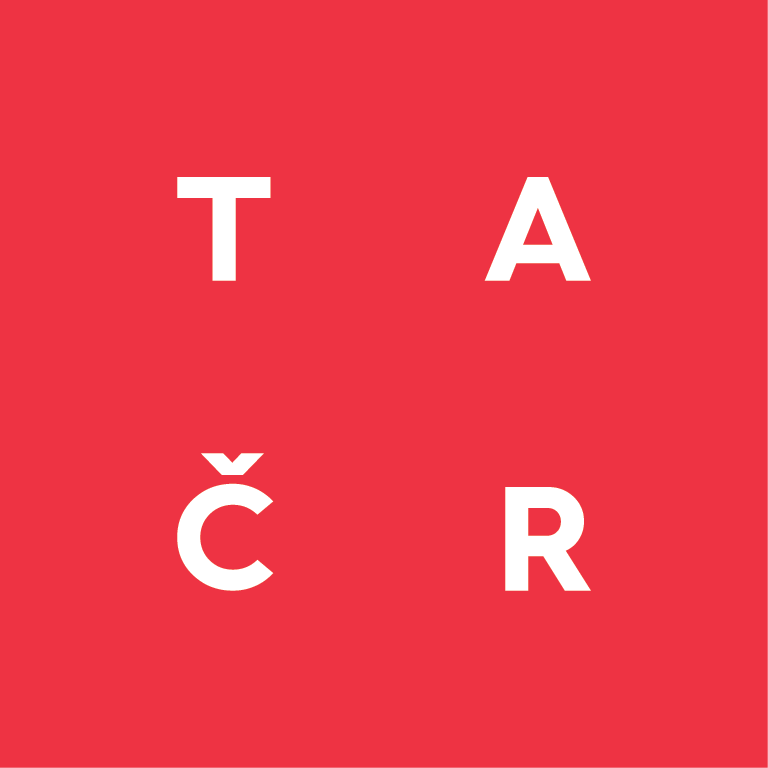03 11 2021

# OEFPIL

## Optimal Estimation of Parameters by Iterated Linearization

The original version of this software was written in R by Stanislav Zámečník, Zdeňka Geršlová and Vojtěch Šindlář in year 2021. The package is based on theoretical background of work of prof. Gejza Wimmer and afterwards implemented by mentioned authors. Main features of the package include:

• estimation of parameters of nonlinear function by iterated linearization
• possibility to use generic functions to OEFPIL objects
• extract confidence bands for set of points
• confidence intervals for parameters
• extract summary of used model
• get covariance matrix for model parameters
• plot the OEFPIL object in a different ways
• plot of estimated curve
• plot of estimated curve with ggplot2 package
• count orthogonal residuals for OEFPIL object
• print out information about OEFPIL object
• calculate estimates of parameters in Nanoindentation
• two datasets from nanoindentation measurements

## Installation

You can install the release version of package from CRAN:

``install.packages("OEFPIL")``

Or the development version from GitHub repository:

``devtools::install_github("OEFPIL/OEFPIL")``

## Usage

In R session do:

``````library(MASS)
steamdata <- steam
colnames(steamdata) <- c("x","y")
k <- nrow(steamdata)
CM <- diag(rep(10,2*k))``````

Creating OEFPIL object which we want to work with

``````library(OEFPIL)
st1 <- OEFPIL(steamdata, y ~ b1 * 10 ^ (b2 * x/ (b3 + x)),
list(b1 = 5, b2 = 8, b3 = 200), CM, useNLS = FALSE)``````

Displaying results using summary function

``summary(st1)``
``````## Summary of the result:
##
## y ~ b1 * 10^(b2 * x/(b3 + x))
##
##     Param Est         Std Dev   CI Bound 2.5 %   CI Bound 97.5 %
## b1   4.487870        1.526903         1.495196          7.480545
## b2   7.188155        1.865953         3.530953         10.845356
## b3 221.837783       99.953658        25.932214        417.743352
##
##  Estimated covariance matrix:
##            b1         b2        b3
## b1   2.331432   2.296195  134.3054
## b2   2.296195   3.481782  184.6313
## b3 134.305405 184.631318 9990.7337
##
##  Number of iterations: 10``````

Plot of estimated function

``plot(st1, signif.level = 0.05, interval = "conf", main  = "Estimated function by iterated linearization")``Ggplot graph of estimated function

``````library(ggplot2)
curvplot.OEFPIL(st1, signif.level = 0.05)````````?OEFPIL``